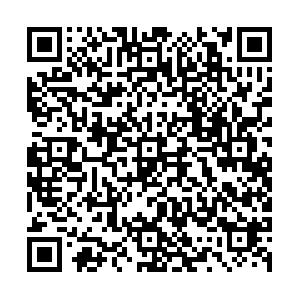# Equal-time kinetic equations in a rotational field• We investigate quantum kinetic theory for a massive fermion system under a rotational field. From the Dirac equation in rotating frame we derive the complete set of kinetic equations for the spin components of the 8- and 7-dimensional Wigner functions. While the particles are no longer on a mass shell in general case due to the rotation-spin coupling, there are always only two independent components, which can be taken as the number and spin densities. With the help from the off-shell constraint we obtain the closed transport equations for the two independent components in classical limit and at quantum level. The classical rotation-orbital coupling controls the dynamical evolution of the number density, but the quantum rotation-spin coupling explicitly changes the spin density.
••Get Citation
Shile Chen, Ziyue Wang and Pengfei Zhuang. Equal-time kinetic equations in a rotational field[J]. Chinese Physics C. doi: 10.1088/1674-1137/ac39fd
Shile Chen, Ziyue Wang and Pengfei Zhuang. Equal-time kinetic equations in a rotational field[J]. Chinese Physics C.Milestone
Article Metric

Article Views(9)
Cited by(0)
Policy on re-use
To reuse of Open Access content published by CPC, for content published under the terms of the Creative Commons Attribution 3.0 license (“CC CY”), the users don’t need to request permission to copy, distribute and display the final published version of the article and to create derivative works, subject to appropriate attribution.
###### 通讯作者: 陈斌, bchen63@163.com
• 1.

沈阳化工大学材料科学与工程学院 沈阳 110142

Title:
Email:

## Equal-time kinetic equations in a rotational field

###### Corresponding author: Ziyue Wang, zy-wa14@mails.tsinghua.edu.cn
• Physics Department, Tsinghua University, Beijing 100084, China

Abstract: We investigate quantum kinetic theory for a massive fermion system under a rotational field. From the Dirac equation in rotating frame we derive the complete set of kinetic equations for the spin components of the 8- and 7-dimensional Wigner functions. While the particles are no longer on a mass shell in general case due to the rotation-spin coupling, there are always only two independent components, which can be taken as the number and spin densities. With the help from the off-shell constraint we obtain the closed transport equations for the two independent components in classical limit and at quantum level. The classical rotation-orbital coupling controls the dynamical evolution of the number density, but the quantum rotation-spin coupling explicitly changes the spin density.

### HTML关注分享DownLoad:  Full-Size Img  PowerPoint